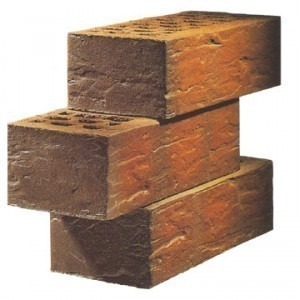•• # Brick Sizes

There are several brick sizes available. When looking for bricks, specify the size rather than the name. Bricks with the same name may have different specs due to differences in the way they’re made.

### Common Brick Dimensions

In the United States, the typical bricks are 8 × 4 × 2.25 inches (203 × 102 × 57 mm) while in Australia they are 9 x 4.33 x 3 inches (230 x 110 x 76mm). In South Africa the measurements are 8.75 x 4 x 3 inches (222 x 106 x 73mm).

### Face Bricks

The Standard’s nominal size is 4" x 2 2/3" x 8" with the actual size at 3 5/8" x 2 ¼" x 8". The number per square feet is 6.27. For the Modular, the nominal brick size is 4" x 2 2/3" x 8" and the actual size is 3 5/8" x 2 ¼" x 7 5/8". The number per sq ft is 6.86.

The nominal size for the King is 3 3/8" x 3" x 10" and the actual size is 3" x 2 5/8" x 9 5/8". The number per square feet is 4.80.

The nominal size for the Queen is 2 ¾" x 3" x 10" and the actual size is 3 1/8" x 2 ¾" x 9 5/8". The number per sq ft is 4.61. For the Engineer, the nominal size is 4" x 3 1/5" x 8". The actual size is 3 5/8" x 2 13/16" x 7 5/8" (number per sq ft is 5.65).

The Economy brick size is 4" x 4" x 8". The actual size is 3 5/8" x 3 5/8" x 7 5/8" and the number per sq ft is 4.50. The Utility face brick has a nominal measurement of 4" x 4" x 12" and an actual size of 3 5/8" x 3 5/8" x 11 ½" (number per sq ft 3.03).

The Jumbo nominal size is 4" x 3" x 8". The actual size is 3 5/8" x 2 ¾" x 8". The number per sq ft is 5.50. The nominal size for the Norman is 4" x 2 2/3" x 12" and the actual size is 3 5/8" x 2 ¼" x 11 5/8". The number per sq ft is 4.57.

For the Norwegian the nominal brick size is 3 ½" x 3" x 12". The actual size is 3 ½" x 2 ¾" x 11 5/8". The number per sq ft is 3.84.

### Brick Quantities

For 1 sq m, the quantity is 60 bricks; for 2 sq m, it is 120 bricks; for 5 sq m, it is 300 bricks and for 10 sq m it is 600 bricks. The mortar quantities are 0.02 cubic meters, 0.05 cubic meters, 0.12 cubic meters and 0.24 cubic meters respectively.

Note: don’t be surprised if brick makers use different figures. In fact, the same type (i.e., Utility) may represent different figures from different manufacturers.

For the most part, choosing brick sizes in the US shouldn’t be hard. As long as you can specify the amount, you’ll get the needed quantity.